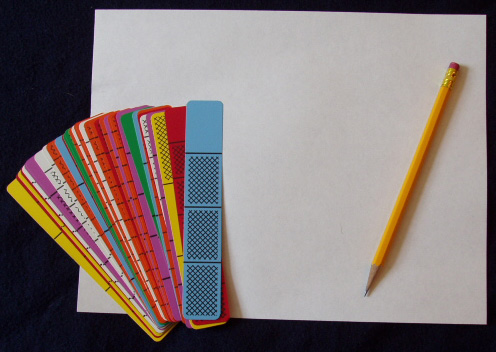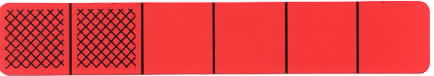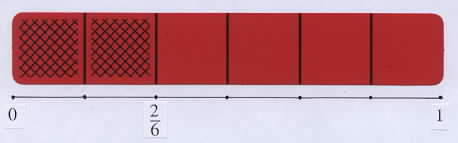Home
 Linear Model - Number Lines Materials: Deck of Fraction Bars per group and one sheet of paper and pencil per personShow a bar for 2/6 and note that the rectangular shape of the bars, as compared to the circular shape of discs or pies, has the advantage that it conveniently extends to a linear model for number lines.Students often experience difficulty locating fractions on a number line. The "Forming A Number Line" activity below shows how the Fraction Bars model provides a natural connection to the number line model. Group Activity: Drawing Number Lines Each person will need a sheet of paper and one of the red bars with 1 to 5 parts shaded for drawing the beginning of a number line. Describe and carry out the following steps as each person forms a number line with their bar. 1. Use the edge of the bar to draw a line segment the length of the bar. Mark seven points on the line, one for each end of the bar and one for each of the five vertical lines of the bar. At the beginning of the bar, label the left end point on the line with 0, and at the end of the bar label the right end point on the line with 1. Point out that the length from 0 to 1 is called a unit length. 2. Each student should write the fraction for the bar beneath the division line and at the end of the shaded amount of their bar. Point out that the end of the shaded amount of the bar indicates where the fraction for the bar should be placed on the line. The use of Fraction Bars can help students understand the correct location when placing fractions on a number line. When students just have the points on the line and not the bars, a common mistake is to begin counting at the zero point, and place the fraction for 2/6 beneath the second point rather than beneath the third point.3. Place the other red bars with different shaded amounts on the line and write their fractions on the line. 4. Use this unit line segment to measure the length of a small object such as a pencil, marker, etc., to the nearest 1/6. Discuss rounding to the nearest fraction on the line. If the length of an object is half way between two points on the number line, such as points for 4/6 and 5/6, we usually round up to the greater fraction.Discuss this activity and the process of measuring and rounding off to the nearest 1/6. To measure longer lengths, the number line can be extended from 1 to 2 by repeating the above steps, and by labeling the division points with the mixed numbers 1 1/6, 1 2/6, etc. Discuss the effect of measuring with a number line that is formed using the orange bars: The measurement will be more precise because of the greater number of division points in the unit. One activity on the Number Lines mats is to move markers along the lines to the end of the mat. The linear nature of the bars is being used on both of the mats below. The two students in the foreground are moving markers on a number lines mat according to the shaded amount of a bar, and the two students in the background are marking progress by placing the shaded amounts of their bars end- to-end on the number lines mat.